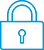cancel
Showing results for
Search instead for
Did you mean:It has been a while since anyone has replied. Simply ask a new question if you would like to start the discussion again.New member
2 1 0 0
Message 1 of 6
1,550
Flag Post

Solved!

# HP Prime - Math errorHP Recommended
HP Prime

When I put the equation 3/(1/3) = 9.00000000001

When I put the pentium FDIV bug test:

(1234/4321)*(4321/1234) = .9999999999

(Thank you Professor Niicely)

Why is this error happening and is there a fix?

Tags (1)
5 REPLIES 5Level 9
971 954 119 186
Message 2 of 6
Flag PostHP Recommended

This is not an error.

This is the expected result when using the approximate numerical domain of the HOME environment.

In the exact CAS (Computer Algebraic System) environment, you will get the results I think you expect, i.e. 9 and 1.

All numerical calculators are approximate, however Some numerical calculators may *seem* to get the exact answers.

See my explanation here:

https://h30434.www3.hp.com/t5/Calculators/sin-pi-not-equaling-0/m-p/6402852#M8604

-Bart
_________________________________________________________
calculator enthusiastLevel 9
971 954 119 186
Message 3 of 6
Flag PostHP Recommended

Further on the Pentium FDIV bug:

https://en.wikipedia.org/wiki/Pentium_FDIV_bug

Quote:

"The presence of the bug can be checked manually by performing the following calculation in any application that uses native floating point numbers, including the Windows Calculator or Microsoft Excel in Windows 95/98.

The correct value is:
4195835/3145727=1.333820449136241002

When converted to the hexadecimal value used by the processor, 4,195,835 = 0x4005FB and 3,145,727 = 0x2FFFFF. The '5' in 0x4005 triggers the fault in the FPU control logic. As a result, the value returned by a flawed Pentium processor is incorrect at or beyond four digits:
4195835/3145727=1.333739068902037589 "

The HP Prime gives the answer of

4195835/3145727=1.33382044914

which is 100% correct to the calculator's 12 digits of precision.

-Bart
_________________________________________________________
calculator enthusiastLevel 9
971 954 119 186
Message 4 of 6
Flag PostHP Recommended

Let's look at (1234/4321)*(4321/1234) a bit further:

1234/4321 = 0.285582041194168016662809534829900485998611432538764174959...

but rounded to the calculator's 12 digits = 0.285582041194

4321/1234 = 3.501620745542949756888168557536466774716369529983792544570...

but rounded to the calculator's 12 digits = 3.50162074554

Now 0.285582041194*3.50162074554 = 0.99999999999856927177476

rounded to the calculator's 12 digits = 0.999999999999

So the calculator is working perfectly within it's 12-digit capability.

Perhaps it would be nice if the calculator had more digits, but how much is enough? and at what point does a calculator become a computer?

-Bart
_________________________________________________________
calculator enthusiast
Tags (1)New member
2 1 0 0
Message 5 of 6
Flag PostHP Recommended

Thank you for the logic and I understand that PI needs some approximation as it is irrational number.

I appricate that CAS provides the true result, So I am not concerned there is a "Chip" error.

I also see the results are true when run with a fixed number format.

I still need a bit more if you could?

As there is still some kind of rounding when the format is "Fixed"

(Also on the TI so they must stop extending and round the number somewhere)

Does anyone know where HP's program extend with "Standard" number formats?

20 places, 50....  (Or do I not really get it).Level 9
971 954 119 186
Message 6 of 6
Flag PostHP Recommended

Hi,

In HOME the Prime is 12 digits. That is the maximum number of digits it will retain for any number. If you use FIX, SCI or ENG notation, only the display is the number is affected, it will still retain all 12 digits. (The only functions that "loose" digits are e.g. "integer part", "fractional part", "round", "truncate" etc.).

However, you may read somewhere that "internally, functions are 15 digits". So what that means is that e.g. the function SIN will calculate to 15 digits, then round the result to 12 digits when it passes out of the function.

This "internally to 15 digits" does not extend outside individual functions,

for example consider a simple formula: SIN(num1)*COS(num2)

once the SIN and COS functions have completed, the two 12 digit results will be multiplied.

However, in CAS things are slightly different. For example it can handle almost infinite long integers (limited by available memory I believe).

For example in CAS do 69! and you'll get a very long number ( in HOME you'll get 1.71122452428ᴇ98 )

When in CAS it is a good idea to use integers, i.e. instead of 12.34 rather enter 1234/100.

For example in CAS, SIN(0.25*PI) gives 0.707106781187

but SIN(PI/4) gives the exact answer of 1/ROOT(2)

Hope this helps.

-Bart
_________________________________________________________
calculator enthusiast
† The opinions expressed above are the personal opinions of the authors, not of HP. By using this site, you accept the Terms of Use and Rules of Participation